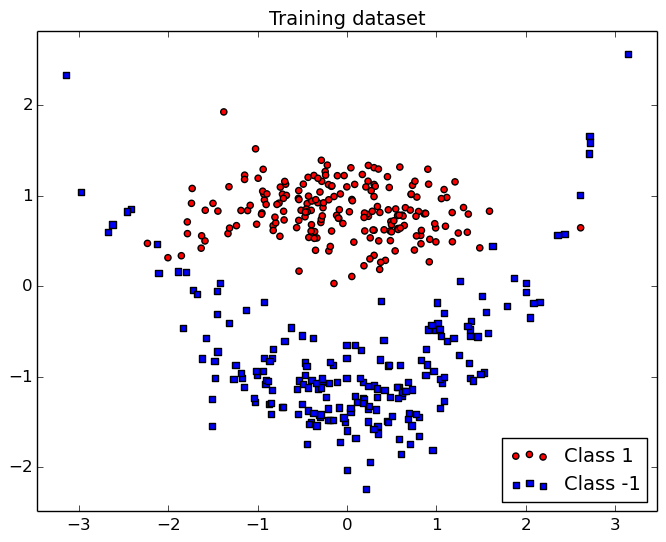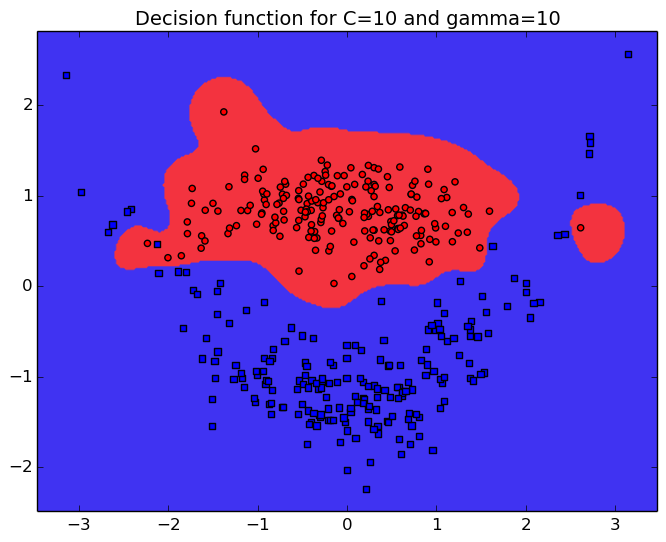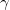# Rémi Flamary

Professional website

## SVM and regularization

$\def\w{\mathbf{w}}$

In this demo, we illustrate the effect of regularization and kernel parameter choice on the decision function of support vector machines (SVM).

### Short introduction to SVM

The decision function of a support vector machine classifier is obtained through the minimization of the following optimization problem:

$$\min_{f}\quad C\sum_{i=1}^{n} \max(0,1-y_i f(\mathbf{x}_i)) + \|f\|^2$$

where C is a regularization parameter that balances the data fitting (left hand term) and the regularization (right hand term). Note that the data fitting term uses a training set $\{\mathbf{x}_i,y_i\}_{i=1\dots n}$ that consists in a list of samples $\mathbf{x}_i\in\mathbb{R}^d$ and their associated class $y_i\in\{1,-1\}$.

One of the strengh of SVM is their ability to chose a complex representation of the data thanks to the use of a kernel function $k(\cdot,\cdot)$ that measures the similarity between samples. The decision function is of the form

$$f(\mathbf{x}) =\sum_{i=1}^n \alpha_i y_i k(\mathbf{x},\mathbf{x}_i)$$

In practice the Gaussian kernel (also known as RBF) defined as

$$k(\mathbf{x},\mathbf{x}')=\exp{(-\gamma \|\mathbf{x}- \mathbf{x}'\|^2)}$$

is often used when the decision function has to handle non-linearities.

When using SVM with a gaussian kernel, one has to select two important parameters: C and $\gamma$. In this demo we illustrate the effect of those parameters on the final decision function of the SVM.

### Dataset used in the demo

In this demo, we illustrate SVM using a 2D non-linear toy dataset also known as "Clown". The main advantage of a 2D example is that it is easy to plot and visualize the samples of each classes in a classical scatter plot figure as shown below.In this Figure, we can see that a non-linear function has to be used for a correct classification but the complexity of the fonction is limited. As illustrated in the next section, the parameters have to be chosen carefully.

### Regularization demo#### Performance

Rec. rate

Current value: C=10

Current value:=10

RR=0.9754

The C parameter that will balance the data and regularization term, which will promote smooth decision function when C is small. The parameter $\gamma$ of the gaussian kernel is also extremely important as it will define the neighborhood of the samples. A large $\gamma$ leads to more complex function. The precision of the classifier on a large test sample is also reported on the right as RR (for recognition rate).

### References

For more information about Support Vector Machine, I strongly recommend  that is a classic introduction. A very good course by Stéphane Canu is also available online .

The figures have been generated using Python,Numpy, and Scikit Learn. The code is avalable here.

 Learning with kernels: support vector machines, regularization, optimization, and beyond, B Scholkopf, AJ Smola, 2001, MIT Press.

 Understanding SVM, S. Canu.